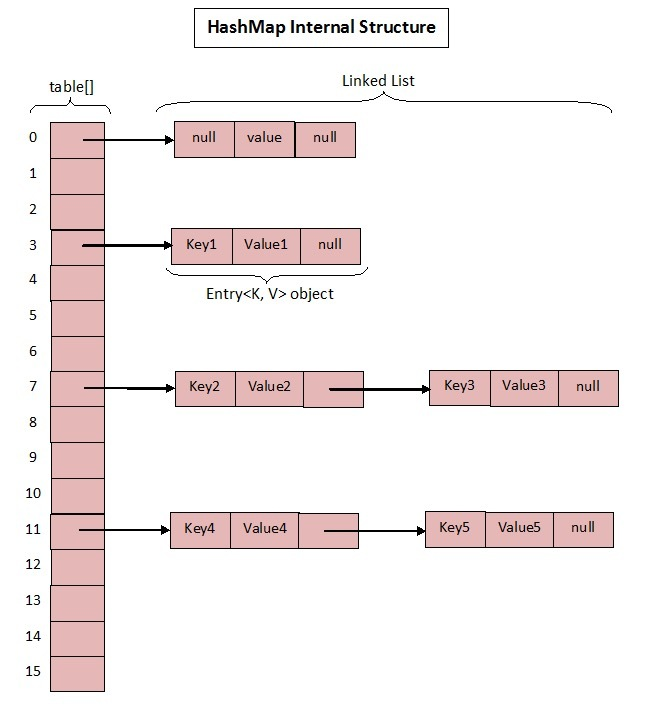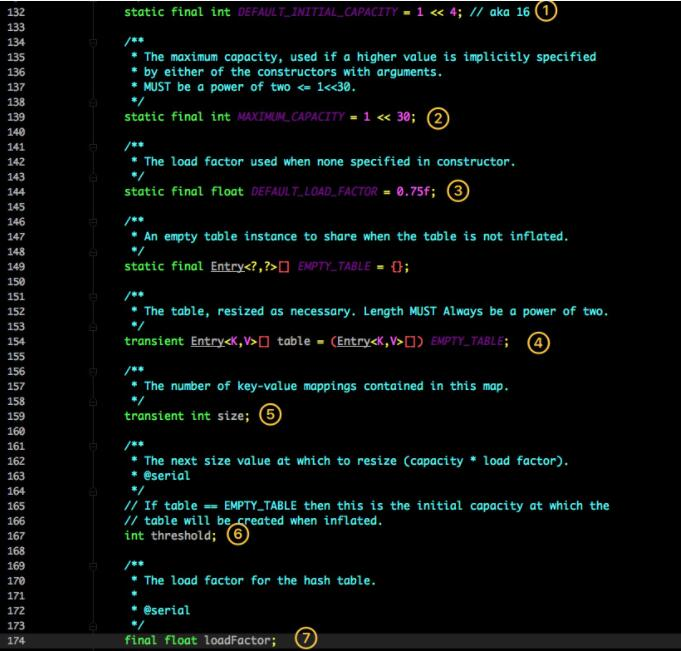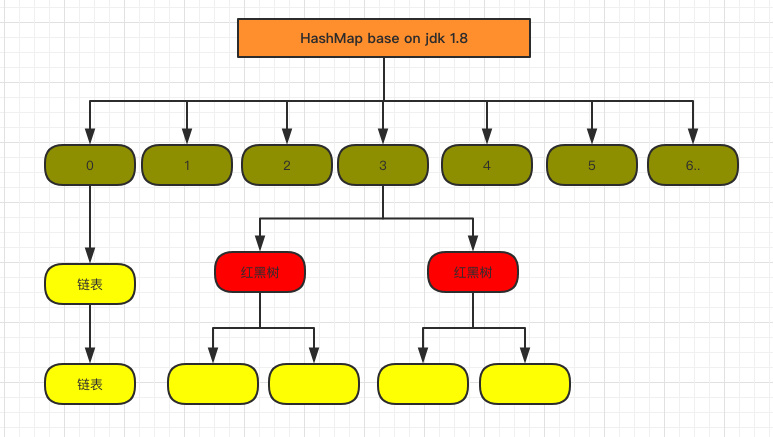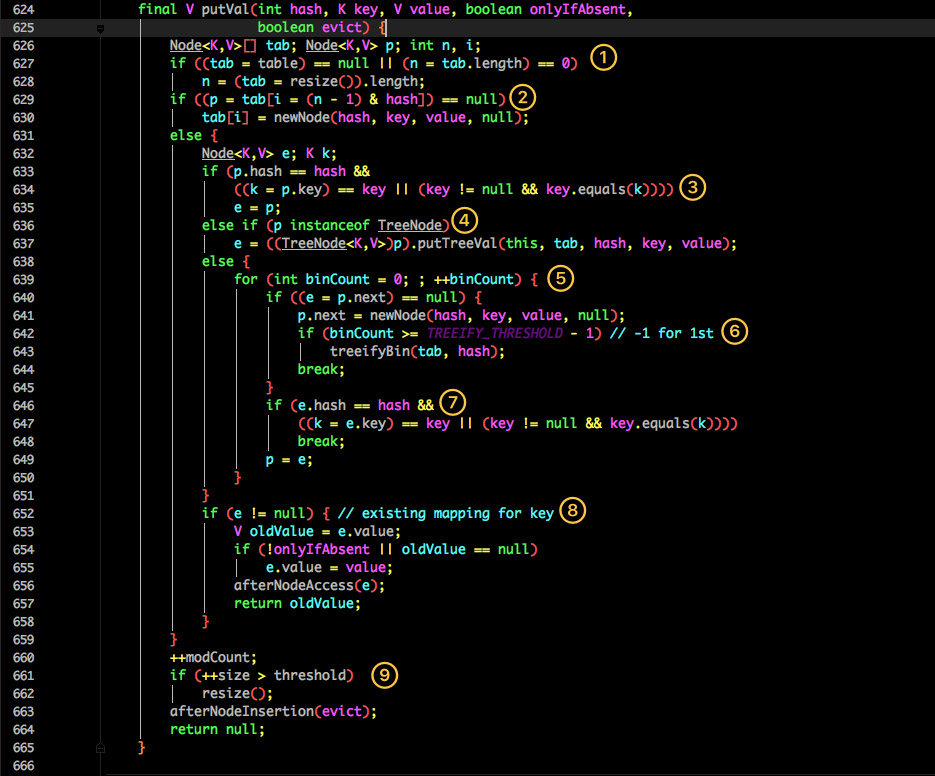# 前言

Map 这样的 `Key Value` 在软件开发中是非常经典的结构，常用于在内存中存放数据。

# jdk 1.7

1.7 中的数据结构图：先来看看 1.7 中的实现。这是 HashMap 中比较核心的几个成员变量；看看分别是什么意思？

1. 初始化桶大小，因为底层是数组，所以这是数组默认的大小。
2. 桶最大值。
3. 默认的负载因子（0.75）
4. table 真正存放数据的数组。
5. Map 存放数量的大小。
6. 桶大小，可在初始化时显式指定。
7. 负载因子，可在初始化时显式指定。

``````public HashMap() {
}
public HashMap(int initialCapacity, float loadFactor) {
if (initialCapacity < 0)
throw new IllegalArgumentException("Illegal initial capacity: " +
initialCapacity);
if (initialCapacity > MAXIMUM_CAPACITY)
initialCapacity = MAXIMUM_CAPACITY;
throw new IllegalArgumentException("Illegal load factor: " +
threshold = initialCapacity;
init();
}
``````

`transient Entry<K,V>[] table = (Entry<K,V>[]) EMPTY_TABLE;`

Entry 是 HashMap 中的一个内部类，从他的成员变量很容易看出：

• key 就是写入时的键。
• value 自然就是值。
• 开始的时候就提到 HashMap 是由数组和链表组成，所以这个 next 就是用于实现链表结构。
• hash 存放的是当前 key 的 hashcode。

## put 方法

``````public V put(K key, V value) {
if (table == EMPTY_TABLE) {
inflateTable(threshold);
}
if (key == null)
return putForNullKey(value);
int hash = hash(key);
int i = indexFor(hash, table.length);
for (Entry<K,V> e = table[i]; e != null; e = e.next) {
Object k;
if (e.hash == hash &　& ((k = e.key) == key || key.equals(k))) {
V oldValue = e.value;
e.value = value;
e.recordAccess(this);
return oldValue;
}
}
modCount++;
return null;
}
``````
• 判断当前数组是否需要初始化。
• 如果 key 为空，则 put 一个空值进去。
• 根据 key 计算出 hashcode。
• 根据计算出的 hashcode 定位出所在桶。
• 如果桶是一个链表则需要遍历判断里面的 hashcode、key 是否和传入 key 相等，如果相等则进行覆盖，并返回原来的值。
• 如果桶是空的，说明当前位置没有数据存入；新增一个 Entry 对象写入当前位置。
``````void addEntry(int hash, K key, V value, int bucketIndex) {
if ((size >= threshold) &　& (null != table[bucketIndex])) {
resize(2 * table.length);
hash = (null != key) ? hash(key) : 0;
bucketIndex = indexFor(hash, table.length);
}
createEntry(hash, key, value, bucketIndex);
}
void createEntry(int hash, K key, V value, int bucketIndex) {
Entry<K,V> e = table[bucketIndex];
table[bucketIndex] = new Entry<>(hash, key, value, e);
size++;
}
``````

## get方法

``````public V get(Object key) {
if (key == null)
return getForNullKey();
Entry<K,V> entry = getEntry(key);
return null == entry ? null : entry.getValue();
}
final Entry<K,V> getEntry(Object key) {
if (size == 0) {
return null;
}
int hash = (key == null) ? 0 : hash(key);
for (Entry<K,V> e = table[indexFor(hash, table.length)];
e != null;
e = e.next) {
Object k;
if (e.hash == hash &　&
((k = e.key) == key || (key != null &　& key.equals(k))))
return e;
}
return null;
}
``````
• 首先也是根据 key 计算出 hashcode，然后定位到具体的桶中。
• 判断该位置是否为链表。
• 不是链表就根据 key、key 的 hashcode 是否相等来返回值。
• 为链表则需要遍历直到 key 及 hashcode 相等时候就返回值。
• 啥都没取到就直接返回 null 。

# jdk 1.8

1.8 HashMap 结构图：先来看看几个核心的成员变量：

``````static final int DEFAULT_INITIAL_CAPACITY = 1 << 4; // aka 16
/**
* The maximum capacity, used if a higher value is implicitly specified
* by either of the constructors with arguments.
* MUST be a power of two <= 1<<30.
*/
static final int MAXIMUM_CAPACITY = 1 << 30;
/**
* The load factor used when none specified in constructor.
*/
static final float DEFAULT_LOAD_FACTOR = 0.75f;
static final int TREEIFY_THRESHOLD = 8;
transient Node<K,V>[] table;
/**
* Holds cached entrySet(). Note that AbstractMap fields are used
* for keySet() and values().
*/
transient Set<Map.Entry<K,V>> entrySet;
/**
* The number of key-value mappings contained in this map.
*/
transient int size;
``````

• `TREEIFY_THRESHOLD` 用于判断是否需要将链表转换为红黑树的阈值。
• HashEntry 修改为 Node。

Node 的核心组成其实也是和 1.7 中的 HashEntry 一样，存放的都是 key value hashcode next 等数据。

## put 方法看似要比 1.7 的复杂，我们一步步拆解：

1. 判断当前桶是否为空，空的就需要初始化（resize 中会判断是否进行初始化）。
2. 根据当前 key 的 hashcode 定位到具体的桶中并判断是否为空，为空表明没有 Hash 冲突就直接在当前位置创建一个新桶即可。
3. 如果当前桶有值（ Hash 冲突），那么就要比较当前桶中的 key、key 的 hashcode 与写入的 key 是否相等，相等就赋值给 e,在第 8 步的时候会统一进行赋值及返回。
4. 如果当前桶为红黑树，那就要按照红黑树的方式写入数据。
5. 如果是个链表，就需要将当前的 key、value 封装成一个新节点写入到当前桶的后面（形成链表）。
6. 接着判断当前链表的大小是否大于预设的阈值，大于时就要转换为红黑树。
7. 如果在遍历过程中找到 key 相同时直接退出遍历。
8. 如果 `e != null` 就相当于存在相同的 key,那就需要将值覆盖。
9. 最后判断是否需要进行扩容。

## get 方法

``````public V get(Object key) {
Node<K,V> e;
return (e = getNode(hash(key), key)) == null ? null : e.value;
}
final Node<K,V> getNode(int hash, Object key) {
Node<K,V>[] tab; Node<K,V> first, e; int n; K k;
if ((tab = table) != null &　& (n = tab.length) > 0 &　&
(first = tab[(n - 1) & hash]) != null) {
if (first.hash == hash &　& // always check first node
((k = first.key) == key || (key != null &　& key.equals(k))))
return first;
if ((e = first.next) != null) {
if (first instanceof TreeNode)
return ((TreeNode<K,V>)first).getTreeNode(hash, key);
do {
if (e.hash == hash &　&
((k = e.key) == key || (key != null &　& key.equals(k))))
return e;
} while ((e = e.next) != null);
}
}
return null;
}
``````

get 方法看起来就要简单许多了。

• 首先将 key hash 之后取得所定位的桶。
• 如果桶为空则直接返回 null 。
• 否则判断桶的第一个位置(有可能是链表、红黑树)的 key 是否为查询的 key，是就直接返回 value。
• 如果第一个不匹配，则判断它的下一个是红黑树还是链表。
• 红黑树就按照树的查找方式返回值。
• 不然就按照链表的方式遍历匹配返回值。

``````final HashMap<String, String> map = new HashMap<String, String>();
for (int i = 0; i < 1000; i++) {
@Override
public void run() {
map.put(UUID.randomUUID().toString(), "");
}
}).start();
}
``````

# 遍历方式

``````Iterator<Map.Entry<String, Integer>> entryIterator = map.entrySet().iterator();
while (entryIterator.hasNext()) {
Map.Entry<String, Integer> next = entryIterator.next();
System.out.println("key=" + next.getKey() + " value=" + next.getValue());
}

Iterator<String> iterator = map.keySet().iterator();
while (iterator.hasNext()){
String key = iterator.next();
System.out.println("key=" + key + " value=" + map.get(key));
}
``````